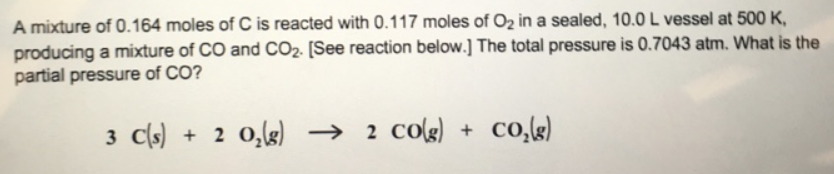# Problem: A mixture of 0.164 moles of C is reacted with 0.117 moles of O2 in a sealed, 10.0 L vessel at 500 K, producing a mixture of CO and CO2. [See reaction below] The total pressure is 0.7043 atm. What is the partial pressure of CO?C(s) + 2 O2(g) → 2 CO(g) + CO2(g)

###### FREE Expert Solution###### Problem Details

A mixture of 0.164 moles of C is reacted with 0.117 moles of O2 in a sealed, 10.0 L vessel at 500 K, producing a mixture of CO and CO2. [See reaction below] The total pressure is 0.7043 atm. What is the partial pressure of CO?
C(s) + 2 O2(g) → 2 CO(g) + CO2(g)